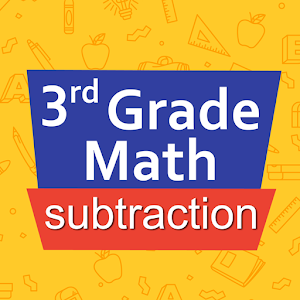Türkçe

Her gün ücretsiz lisanslı Android uygulamaları ve oyunlar sunuyoruz.2.99
Bugün bedava

# Android Giveaway of the Day - Third grade Math - Subtraction

An assistant and training suite for subtraction.
2.99 Bugün bedava
Kullanıcı puanı: 2

sizin için Giveaway of the day farkıyla bedava!
Bu programı indirmek ve kurmak için sınırlı zamanınız var.Intuitive interface powered by handwriting input and a fun and engaging mini game in addition to a regular math trainer mode make our app stand out from the crowd of generic math learning apps.

With Third grade Math - Subtraction you can practice and improve the following math skills:
- Subtraction facts - numbers up to 100
- Subtract two two-digit numbers
- Subtract two three-digit numbers
- Complete the subtraction sentence up to three digits
- Subtract numbers with four or five digits
- Balance subtraction equations up to two digits
- Balance subtraction equations up to three digits

Sergey Malugin

Education

### Sürüm:

Varies with device

### Boyut:

Varies with device

Everyone

### Uyumluluk:

Varies with device

## Third grade Math - Subtraction üzerine yorumlar

Thank you for voting!